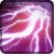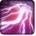# Lightning StormConditions

Used by

## Related effects, buffs and debuffs

Please click on an effect below to view its details.

• [does not expire]Lightning Storm

 Slot: Buff Duration: passive Tick rate: 1s # occurrences: 0
• When effect ticks

Perform the following actions:

• Add effect #2 to TARGET from TARGET
• [0s] [not displayed]Effect #2

 Slot: Buff Duration: 0s Tick rate: does not tick # occurrences: 0 Conditions: Can only by called by other effects
• On Apply
• [target override]

Only when the following conditions are met:

• <
• If PRIMARY TARGET is alive
AND
• If PRIMARY TARGET is a player
>

Perform the following actions:

• Add effect #3 to TARGET from TARGET
• [0s] [not displayed]Effect #3

 Slot: Buff Duration: 0s Tick rate: does not tick # occurrences: 0 Conditions: Can only by called by other effects
• On Apply

Perform the following actions:

• Unknown (183)
- Unknown (303) = (bool) true
- Unknown (306) = (bool) true
- Unknown (430) = (bool) false
- Unknown (310) = (string) aoePositionOptionType_Entity
- Unknown (311) = (string) aoeTarget_Target
- Unknown (313) = (string) aoeScalarType_RandomValue
- Unknown (317) = (string) aoeScalarType_LiteralValue
- Unknown (321) = (string) aoeScalarType_RandomValue
- Unknown (325) = (string) aoeOrientationOptionType_EntityFacing
- Unknown (326) = (string) aoeTarget_Target
- Unknown (344) = (string) aoeSphere
- Unknown (349) = (string) aoeTargetCondition_IsPlayer;aoeTargetCondition_IsAlive
- Unknown (353) = (string) aoeScalarType_LiteralValue
- Unknown (354) = (string) aoeScalarType_LiteralValue
- Unknown (355) = (string) aoeScalarType_LiteralValue
- Unknown (367) = (string) aoeScalarType_LiteralValue
- Unknown (384) = (string)
- Unknown (386) = (string) aoeAbilityCaster_Caster
- Unknown (387) = (string) aoeAbilityTarget_Target
- Unknown (388) = (string) flashpoint/corellia/republic/enemy/boss_3/lightning_ground_target
- Unknown (389) = (string) flashpoint/corellia/republic/enemy/boss_3/lightning_storm_impact
- Unknown (431) = (string) aoeRotation_None
- Unknown (541) = (string) aoeVfxEntity_AreaEffect
- Unknown (545) = (string) aoeVfxEntity_AreaEffect
- Unknown (551) = (string) aoeVfx_PlayAtPosition
- Unknown (552) = (string) aoeVfx_PlayAtPosition
- Ability Spec = (int) 16141070857096540380
- Unknown (307) = (int) 1
- Unknown (308) = (int) 1
- Tick Rate = (float) 1
- Unknown (304) = (float) 3
- Unknown (305) = (float) 3
- Unknown (315) = (float) 0
- Unknown (316) = (float) 0.2
- Unknown (318) = (float) 0
- Unknown (323) = (float) 0
- Unknown (324) = (float) 0.2
- Unknown (362) = (float) 0
- Unknown (363) = (float) 0
- Unknown (364) = (float) 0
- Unknown (368) = (float) 0.25
- Unknown (390) = (float) 0
- Unknown (398) = (float) 2.5